# How to Create Stats in Polygons

Another interesting feature is under the button Special – Stats in Polygons. Using this feature, you can create statistical analysis and summaries from one map layer of polygons to a second layer. An example is shown in the following figure. One polygon layer displays districts and the second contains springs localized in the area of interest with data stored in the attribute table; such as water temperature, mineralization or the yield of the water sources. The column named T_Kat contains information of the spring’s categorization; either above or below 10°C; and this parameter sets all points in the Map Panel in the appropriate color for < 10 °C and > 10 °C.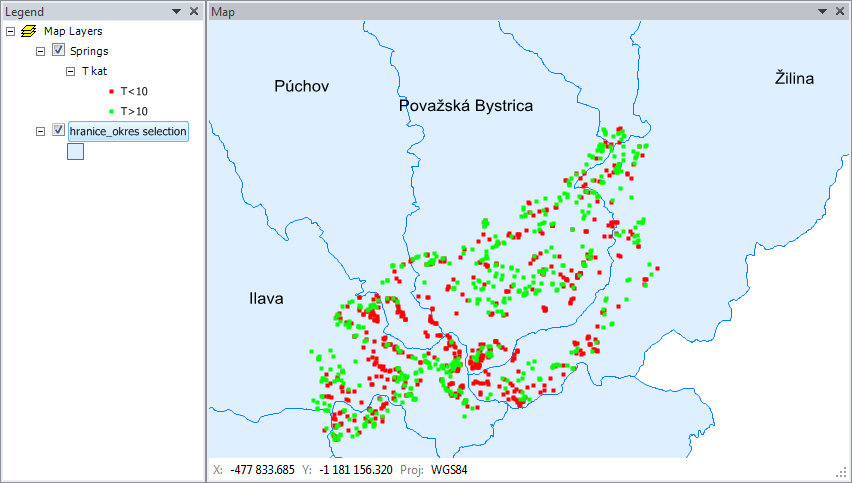You can use Special – Stats in Polygons for the following imagined function. Imagine you want to find the summary of yields for all springs in individual districts, and want to calculate this sum individually for springs with temperature < 10 °C and springs with temperature > 10 °C. Now click Special – Stats in Polygons to display the following dialog.Here, you define your parameters. First choose the Stats for Layer that contains district polygons which delineate the areas. Then define the column from the attribute table that indentifies the individual districts, such as the name of the district. Choose the layer you want to calculate your statistics from in Data Layer. In this example springs are stored in the layer named "Springs". If you want to calculate the yield statistics for all the springs in the district, leave the Group By combo-box empty. But, if you want to calculate the sum of yields individually for the < 10 °C and > 10 °C, put T_kat in the Group By combo-box.

In the Stat Mode combo-box you have a choice "Attribute Data" or "Spatial Data". If you want to calculate statistics based on the data stored in attribute table, select the Attribute Data option; and then select the column you want to use for statistics in the Processing Column combo-box. In this imaginary case, spring yields are stored in column Q. Finally, select the processing function. Next, choose the Sum function because you want the summary yield. After defining all these parameters, click Apply and addin will compute your stastistical parameters. If you have a lot of data in the layers, this may take longer to process. The next message box informs you that your statistics results will be saved to the clipboard; and you can paste the result into the spreadsheet using the Ctrl+V command.The example result is shown in the figure below. The Item column contains your district names which identify your polygons. When you define the Group By combo-box, the Group identification is listed in the next column. Finally, the selected statistical parameter is in the last column under Value. The following example automatically counted the springs yields in individual districts and individually summed values for springs with temperatures above and below 10°C.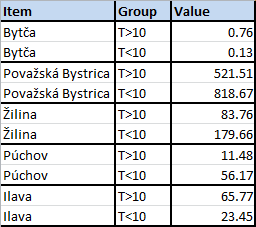The next example for your statistical analyses uses the spatial characteristics of layer features and the same district polygons (instead of values from the attribute table layer used in the previous example). The statistical parameters are computed from the line layer that defines the area’s river network.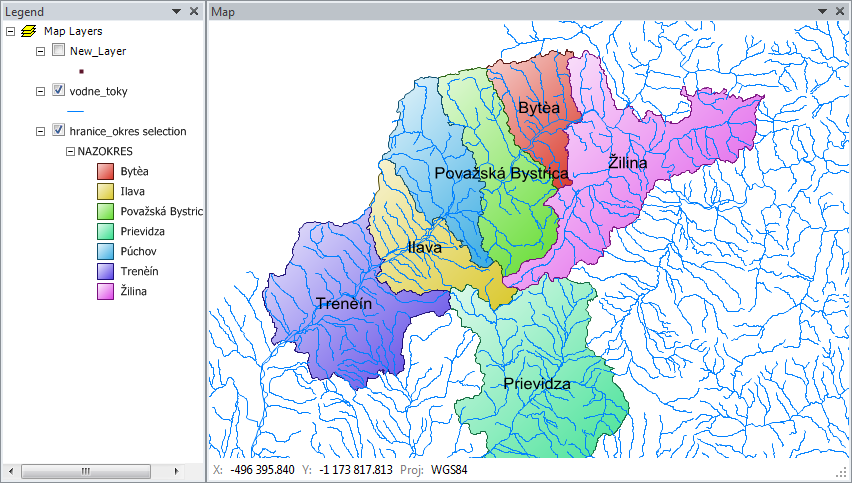Your imagined task here is to calculate the lengths of all rivers and streams in individual districts. Select the layer for computing vodne_toky (= "water-flows") in the Data Layer combo-box for your river network. There are no "groups of rivers" so leave the Group By combo-box empty. Select the Spatial Data item in the Stat Mode combo-box which enables you to calculate the spatial characteristics directly from individual features. Then choose the Length item in the Function combo-box, and this automatically detects the length of each feature in your polygons. Finally click Apply, and add-in calculates the desired river/stream length values and save them to the clipboard.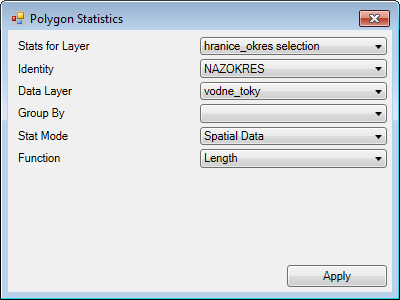Pressing Ctrl+V keys then pastes the following table to the Excel spreadsheet.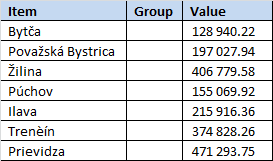The Item column lists your districts. The Group column remains empty, and the last column contains the summary length of rivers and streams in each district. Be careful of the coordinate system you used here; because if you used WGS84, the length values are in degrees, and the metric coordinate system gives the lengths in meters. This described spatial analysis can be performed on lines, points and polygons; and you can calculate lengths, centroid coordinates, areas and the total number of features in the defined polygons.